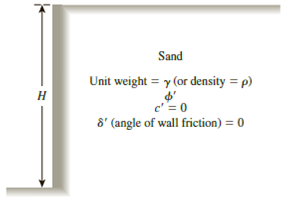Chapter 13, Problem 13.1PPrinciples of Geotechnical Enginee...

9th Edition
Braja M. Das + 1 other
ISBN: 9781305970939

Solutions

Chapter
SectionPrinciples of Geotechnical Enginee...

9th Edition
Braja M. Das + 1 other
ISBN: 9781305970939
Textbook Problem

13.1 through 13.4 Figure 13.39 shows a retaining wall that is restrained from yielding. For each problem, determine the magnitude of the lateral earth force per unit length of the wall. Also, find the location of the resultant, z ¯ , measured from the bottom of the wall.Figure 13.39

To determine

Find the magnitude of the lateral earth forces Po per unit length of the wall and find the location z¯ of the resultant measured from the bottom of the wall.

The magnitude of the lateral force is 393.1kN/m_.

The location of the resultant measured from the bottom of the wall is 2.5m_.

Explanation

Given information:

The height (H) of the retaining wall is 7.5 m.

The soil friction angle ϕ is 31°.

The angle of wall friction δ is 0.

The unit weight γ of the sand is 19.2kN/m3.

The over-consolidation ratio (OCR) is 2.2.

Calculation:

Calculate the earth pressure coefficient (Ko) using the relation.

Ko=(1sinϕ)(OCR)sinϕ

Substitute 31° for ϕ and 2.2 for OCR.

Ko=(1sin31°)(2.2)sin31°=0.48496×1.5009=0.7279

Calculate the total force per unit length (Po) using the relation.

Po=12KoγH2

Substitute 0.7279 for Ko, 19.2kN/m3 for γ, and 7.5m for H.

Po=12×0.7279×19.2×(7.5)2=393.1kN/m

Thus, the magnitude of the lateral earth force per unit length (Po) is 393.1kN/m_.

Calculate the location of the resultant (z¯) using the relation.

z¯=H3

Substitute 7.5m for H.

z¯=7.53=2.5m

Thus, the location of the resultant (z¯) measured from the bottom of the wall is 2.5m_.

The Solution to Your Study Problems

Bartleby provides explanations to thousands of textbook problems written by our experts, many with advanced degrees!

Get Started

Find more solutions based on key concepts